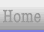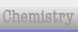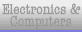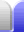#### Area explained

Area is measured in square metres (m2).

It is important to realise that m are not the same as m2.

The area of a triangle is half the area of the smallest rectangle that will enclose it, and so the area of the triangle is half the area of that rectangle.

To find the area of a circle we need to use pi. The area of a square with each side of length r will have area r2. The proportion of that square covered by a quarter of a circle happens to work out to π/4, so the area of the quarter circle is π/4 times r2. This means a whole circle's area is πr2.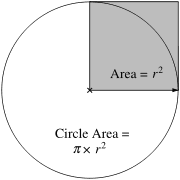### Calculating Area

#### Area of a rectangle:

base x height
[or] length x width

#### Area of a triangle:

1/2 base x height

π·r2

4·π·r2

### Random facts – surface area of humans

An average adult woman has a surface area of about 1.6 m2, while an average adult man has a surface area about 1.8 m2.

The lungs have a greater surface area – about 75 m2. The intestines have even more – their total length is only about 7.5-8.5 m but inside them they have an enormous number of tiny projections called villi (which are in turn covered in microvilli), giving them a total surface area of as much as 200 m2.Home Astronomy Chemistry Electronics Mathematics Physics Field Trips Turn on javascript for email link